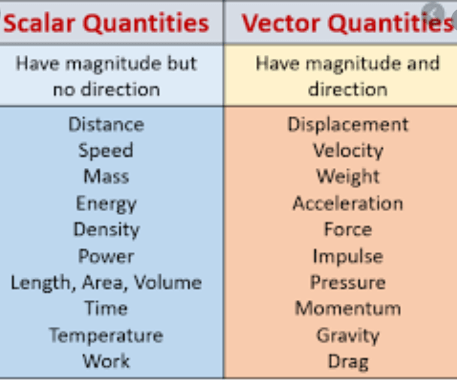Mechanics

# Difference between Vectors and ScalarsThe basic difference between vectors and scalars is that scalar quantity is described completely by its magnitude only while vector quantity is described by magnitude and direction. Speed, energy, power are a few scalar quantity examples. Force, velocity, and acceleration are some vector quantity examples.
Now, we learn in detail about scalar and vector quantities.

## Difference between Vectors and Scalars in Tabular form

 Scalars vectors Physical quantities have magnitude only but no direction. Physical quantities have magnitude and direction. These quantities are completely described by: 1:a number 2:a suitable unit These quantities are completely described by: 1:a numbers 2:by a suitable unit 3:By certain direction These quantities are added, subtracted, multiplied, and divided by simple arithmetic rules. These quantities cannot be added, subtracted, multiplied, and divide by simple arithmetical rules.

### What are some examples of scalar quantity?

• Speed
• work
• distance
• energy
• power
• Temperature
• Volume
• Specific heat
• Calorie
• Density
• Entropy
• Gravitational potential
• Charge
• Frequency
• Kinetic energy

### Examples of vector quantities

• Force
• Acceleration
• Momentum
• Torque
• Electric flux
• Magnetic field intensity
• Centrifugal force
• Shearing stress
• Electric field intensity

• Mass
• weight
• speed
• work
• power
• energy
• force
• torque
• displacement
• distance

### Related Articles

1.rajesh kumar says: